### Introduction

This tutorial shows how you can use promor to analyse label-free quantification (LFQ) proteomics data that do NOT contain technical replicates.

We recommend that you first go through the simple working example provided in Introduction to promor to get acquainted with promor's functionality.

vignette("intro_to_promor")

A tutorial for proteomics data containing technical replicates is provided here: promor: Technical replicates

For this tutorial we will be using a previously published data set from Cox et al. (2014).

### Workflow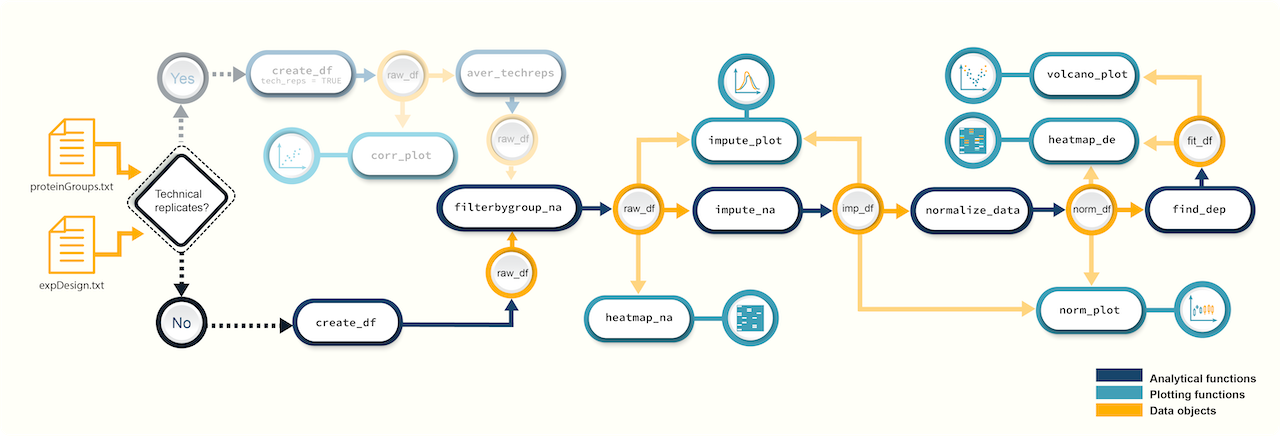Figure 1. A schematic diagram highlighting the suggested promor workflow for proteomics data without technical replicates

You can access the help pages for functions shown above and more using ?function_name

### Input data

To run promor, you need:

• A proteinGroups.txt file produced by MaxQuant or a standard input file containing a quantitative matrix of protein intensities.
• An expDesign.txt file that contains the experimental design.

proteinGroups.txt is one of the output files generated by MaxQuant program. It is a tab-delimited file that contains information on identified proteins from your peptide data. More information on its contents can be found in MaxQuant Documentation.

Standard input file should be a tab-delimited text file. Proteins or protein groups should be indicated by rows and samples by columns. Protein names should be listed in the first column and you may use a column name of your choice for the first column. The remaining sample column names should match the sample names indicated by the mq_label column in the expDesign.txt file.

expDesign.txt file is a tab-delimited text file that contains the design of your experiment. Note that you will have to create and provide this file when you run promor with your own data. Let’s take a look at the sample expDesign.txt file that we will be using for this tutorial.

# Load promor
library(promor)
# Let's load the expDesign.txt file
exp_design <- read.csv("https://raw.githubusercontent.com/caranathunge/promor_example_data/main/ed1.txt", sep = "\t")
# Take a peek inside the file
head(exp_design)

mq_label is the sample label that you provided when running MaxQuant. You can easily find them in your proteinGroups.txt file. Hint: In LFQ intensity columns, the term “LFQ intensity” is immediately followed by the label you provided when running MaxQuant. This is your mq_label. If you are using a standard input file instead of a proteinGroups.txt file, mq_label should match the column headers of the sample columns.

condition is the condition or group that your samples belong to. In this example “H” refers to HeLa cells, and “L” refers to E. coli cells.

sample_ID is a unique identifier for each sample.

tech_rep is a number denoting the technical replicate number. In this example, there are no technical replicates, so the column is left blank.

### 1. Create a raw_df object

Let’s first create a raw_df object with the input files. Here we are using a proteinGroups.txt file and an expDesign.txt file. The default intensity data type to use is “LFQ”, so LFQ intensity columns are extracted from the proteinGroups.txt file.

# Create a raw_df object with default settings.
raw <- create_df(
prot_groups = "https://raw.githubusercontent.com/caranathunge/promor_example_data/main/pg1.txt",
exp_design = "https://raw.githubusercontent.com/caranathunge/promor_example_data/main/ed1.txt"
)
#> 0 empty row(s) removed.
#> 0 empty column(s) removed.
#> 80 protein(s) (rows) only identified by site removed.
#> 65 reverse protein(s) (rows) removed.
#> 42 protein potential contaminant(s) (rows) removed.
#> 1923 protein(s) identified by 2 or fewer unique peptides removed.
#> Zeros have been replaced with NAs.
#> Data have been log-transformed.

# We can quickly check the dimensions of the data frame
dim(raw)
#>  4584    6

Instead of using LFQ intensity values, you can choose to extract other data types such as iBAQ from the proteinGroups.txt file as follows:

# Create a raw_df object of ibaq values
raw_1 <- create_df(
prot_groups = "https://raw.githubusercontent.com/caranathunge/promor_example_data/main/pg1.txt",
exp_design = "https://raw.githubusercontent.com/caranathunge/promor_example_data/main/ed1.txt",
data_type = "iBAQ"
)
#> 0 empty row(s) removed.
#> 0 empty column(s) removed.
#> 80 protein(s) (rows) only identified by site removed.
#> 65 reverse protein(s) (rows) removed.
#> 42 protein potential contaminant(s) (rows) removed.
#> 1923 protein(s) identified by 2 or fewer unique peptides removed.
#> Zeros have been replaced with NAs.
#> Data have been log-transformed.

# We can quickly check the first five rows of the data frame
head(raw_1)

Instead of using a proteinGroups.txt file, you can choose to use a standard input file containing a quantitative matrix of protein intensities.

# Create a raw_df object using a standard input file.
raw_2 <- create_df(
prot_groups = "https://raw.githubusercontent.com/caranathunge/promor_example_data/main/st.txt",
exp_design = "https://raw.githubusercontent.com/caranathunge/promor_example_data/main/ed1.txt",
input_type = "standard"
)
#> 0 empty row(s) removed.
#> 0 empty column(s) removed.
#> Zeros have been replaced with NAs.
#> Data have been log-transformed.

#check the first five rows of the data frame
head(raw_2)

If you ran create_df with default settings using either type of input files, now your raw_df object contains log2 transformed protein intensity values. Run ?create_df for more information on available arguments.

### 2. Filter proteins by groupwise missing data

Next, we will remove proteins if they have more than 0.4% (default is 0.33%) missing data in either group (We will be using the raw_df object that we created with the default settings as input).

# Filter out proteins with high levels of missing data in either condition
raw_filtered <- filterbygroup_na(raw, set_na = 0.4)
#> 224 proteins with higher than 40% NAs in at least one group removed.

# We can check the dimensions of the new data frame. Note that the number of rows have changed.
dim(raw_filtered)
#>  4360    6

### 3. Visualize missing data

Now, we are going to see how missing data is distributed in the data. This will help us make an informed decision on how to impute missing data in the next step.

# Visualize missing data in a subset of proteins.
heatmap_na(raw_filtered, palette = "mako")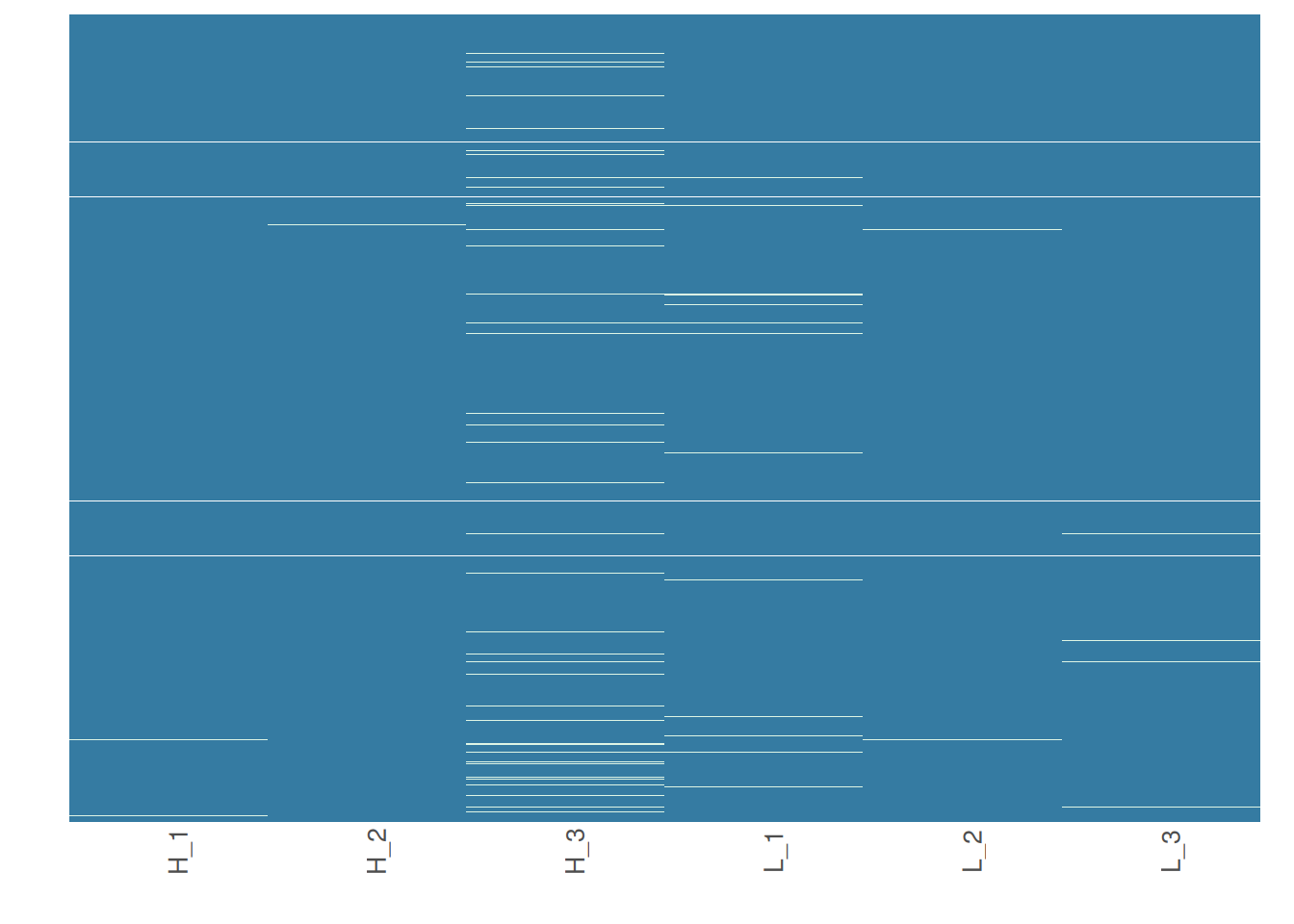Note that your proteins are alphabetically ordered by default, but if you would rather order them by the mean intensity to check if proteins with low intensities are more likely to have missing data, you can do the following:

# Order proteins by mean intensity.
heatmap_na(raw_filtered, reorder_y = TRUE, palette = "mako")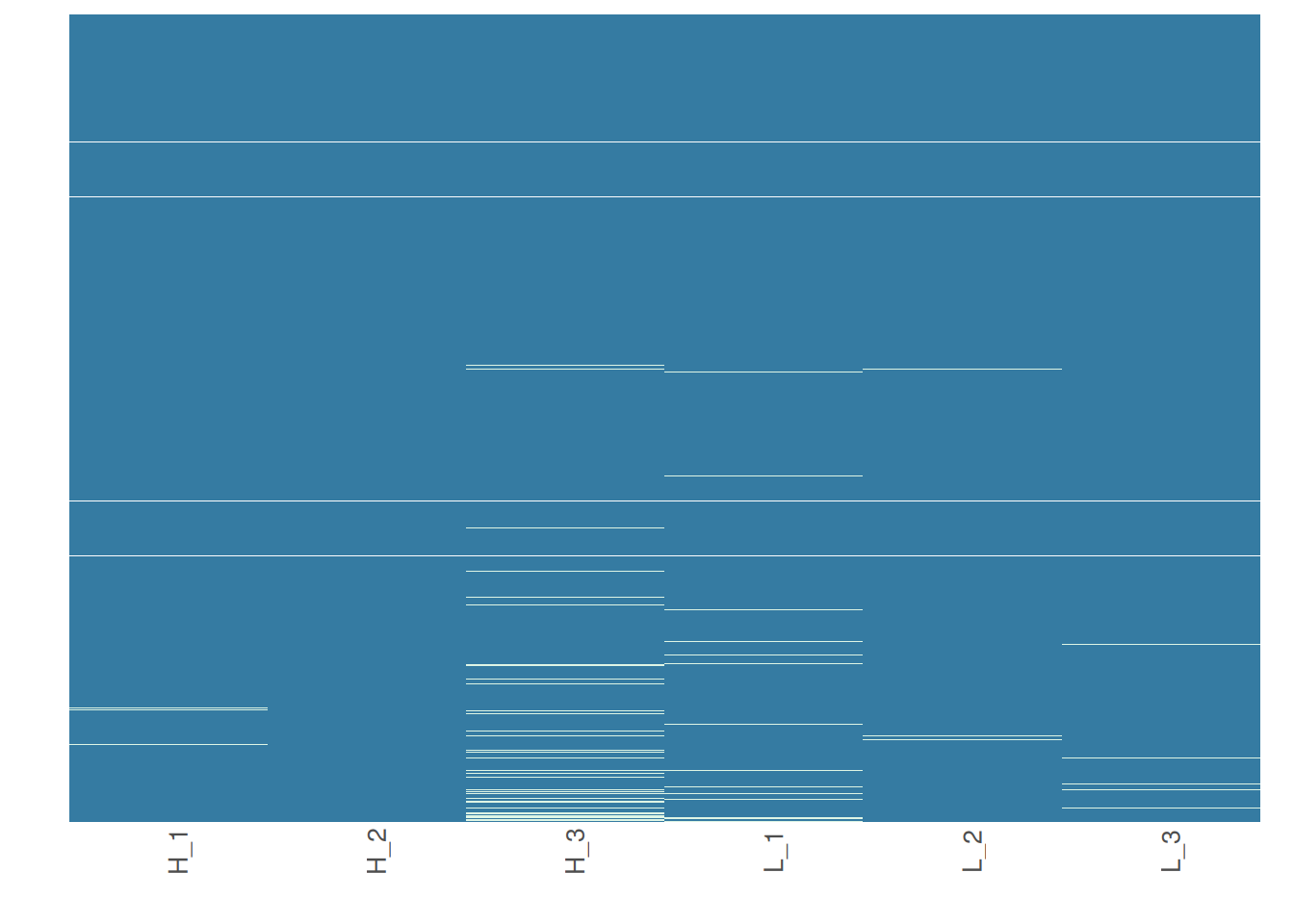For larger data sets, you can choose to visualize subsets of the data

# Visualize missing data in a subset of proteins.
heatmap_na(raw_filtered, protein_range = 40:70, label_proteins = TRUE, palette = "mako")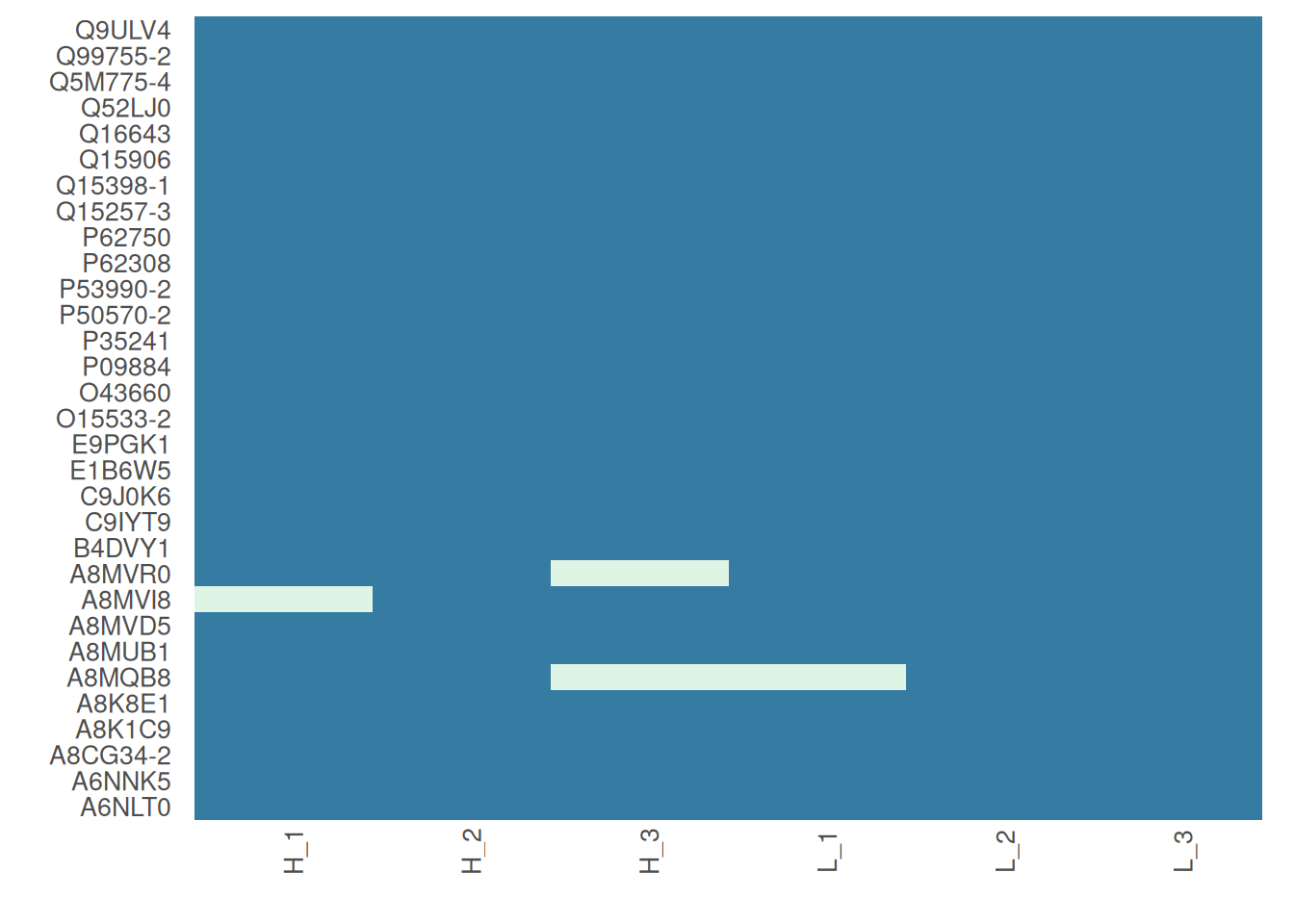### 4. Impute missing data

promor provides multiple missing data imputation methods. Default is minProb, which assumes that all missing data is MNAR (Missing Not at Random) type or left-censored missing values, and imputes them by random draws from a Gaussian distribution centered at a minimal value. More information about available imputation methods can be found in Lazar et al. (2016).

# Impute missing data with minProb method. Don't forget to fix the random seed for reproducibility.
imp_df_mp <- impute_na(raw_filtered, seed = 327)

Alternatively, we can use a different imputation method as follows.

# Impute missing data with minDet method. Don't forget to fix the random seed for reproducibility.
imp_df_md <- impute_na(raw_filtered, method = "minDet", seed = 327)

Note: Some missing data imputation methods such as ‘kNN’ require that the data is normalized prior to imputation.

### 5. Visualize imputed data

We can now visualize the impact of imputation on the data set. Lets check the data imputed with minProb method.

# Visualize the imputed data with sample-wise density plots.
impute_plot(original = raw_filtered, imputed = imp_df_mp, n_row = 3, n_col = 3, palette = "mako")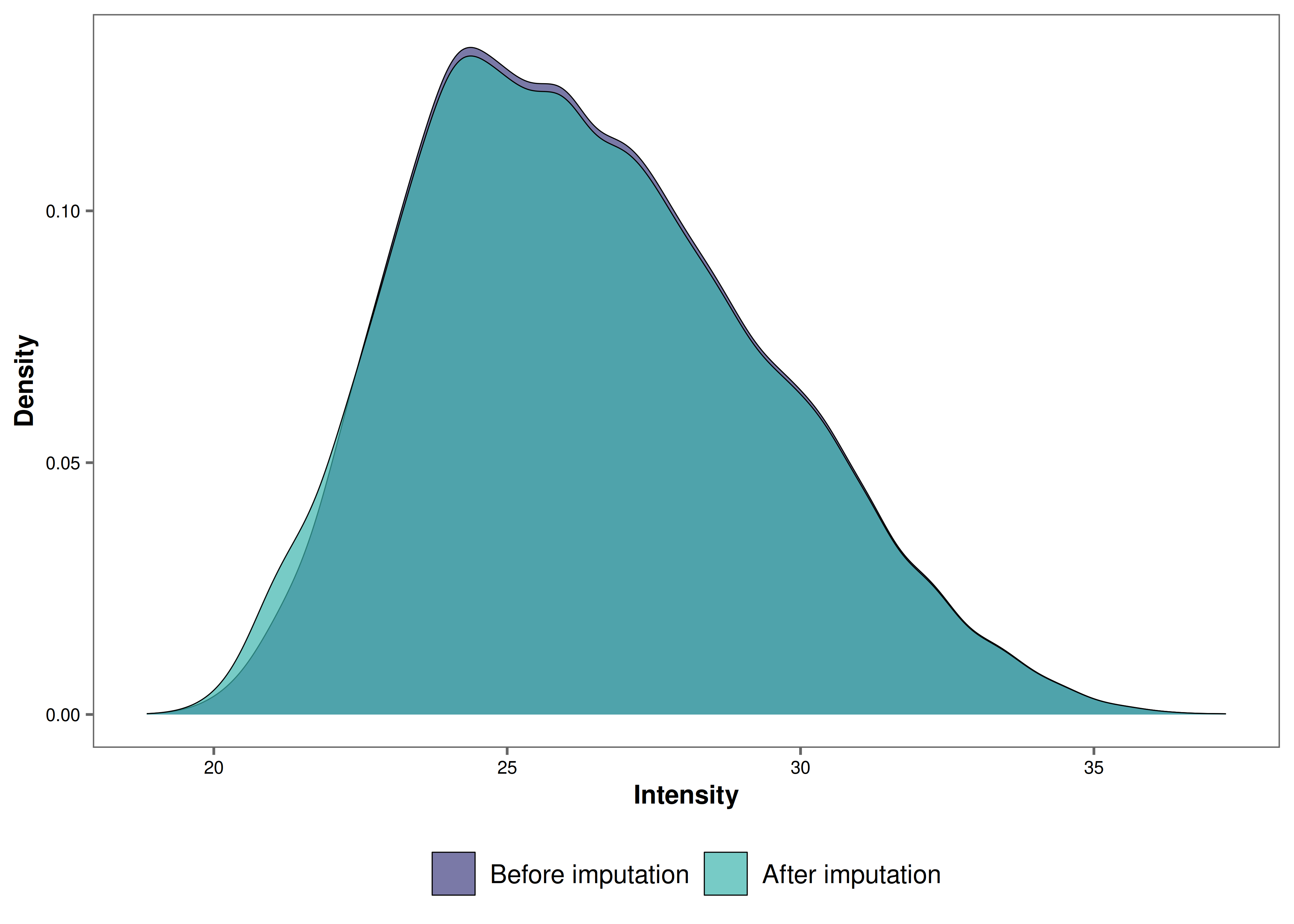We can also visualize the impact of imputation on each sample’s intensity data by setting global = FALSE.

# Visualize the imputed data with sample-wise density plots.
impute_plot(original = raw_filtered, imputed = imp_df_mp, global = FALSE, n_row = 3, n_col = 3, palette = "mako")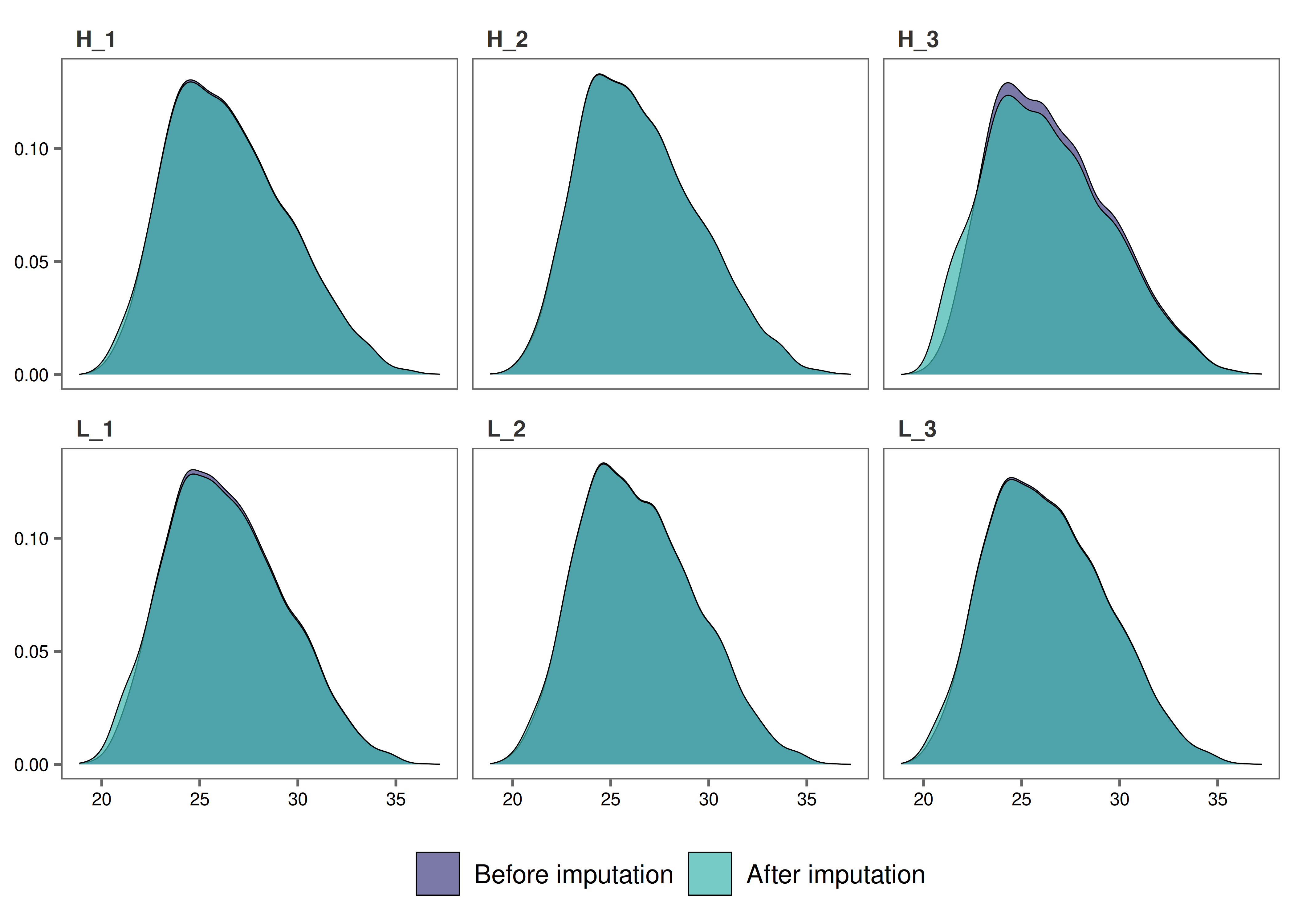It looks like, for most samples, imputation has not changed the distribution of data by much, which is a good sign.

### 6. Normalize data

Note: As MaxQuant has already normalized protein intensities using the MaxLFQ algorithm, further normalization may not be necessary for this data set. Steps 6 and 7 are performed for visualization purposes only.

To normalize the imputed data with the default quantile method:

norm_df <- normalize_data(imp_df_mp)

### 7. Visualize normalized data

Let’s check the results from quantile normalization.

norm_plot(original = imp_df_mp, normalized = norm_df, palette = "mako")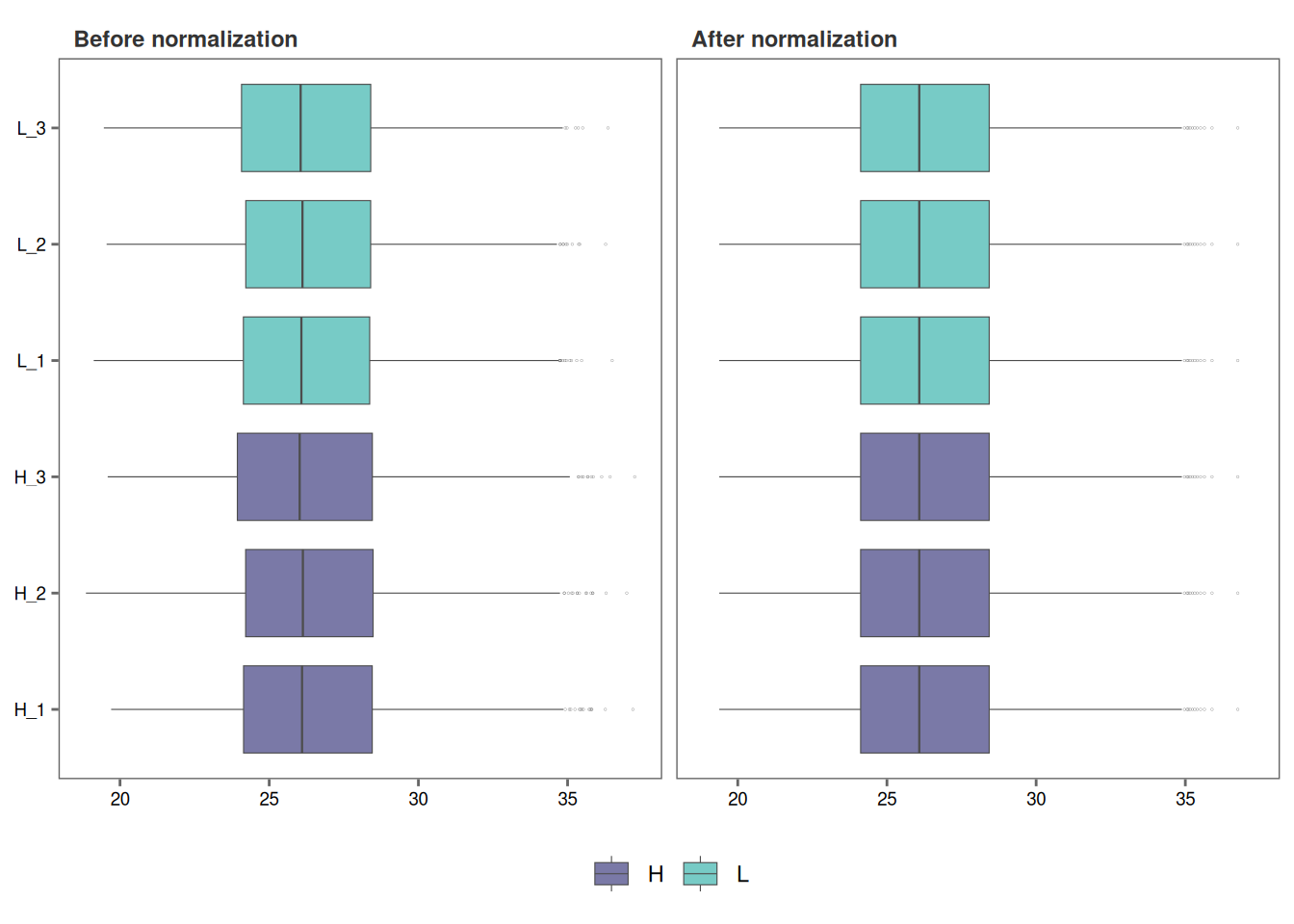For samples that show very little variability among them, such as those in this data set, it may be difficult to visualize the effect of normalization with boxplots (Remember, this data set had been already normalized with the MaxLFQ algorithm within the MaxQuant program). Let’s use type = "density to visualize the effect of normalization with density plots instead.

norm_plot(original = imp_df_mp, normalized = norm_df, type = "density", palette = "mako")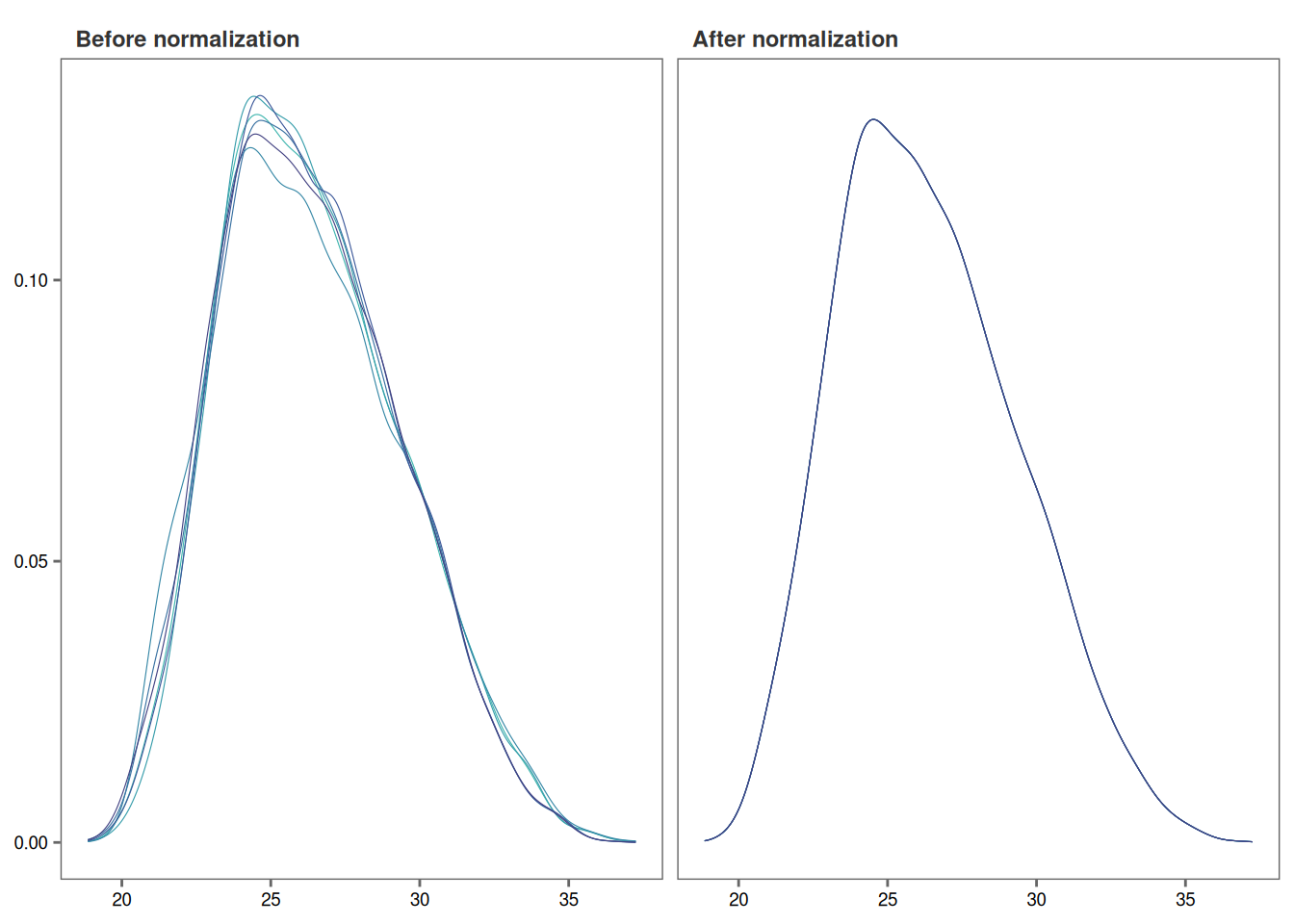### 8. Identify differentially expressed proteins

We will be using the non-normalized imp_df object we created in Step 4 to perform differential expression analysis.

fit_df <- find_dep(imp_df_mp)
#> 1294 siginificantly differentially expressed proteins found.

You can also choose to save the top 10 hits in a text file as follows:

fit_df <- find_dep(imp_df_mp, save_tophits = TRUE, n_top = 10)

### 9. Generate a volcano plot

Let’s visualize the results from Step 8 using a volcano plot.

volcano_plot(fit_df,
text_size = 5,
palette = "mako"
)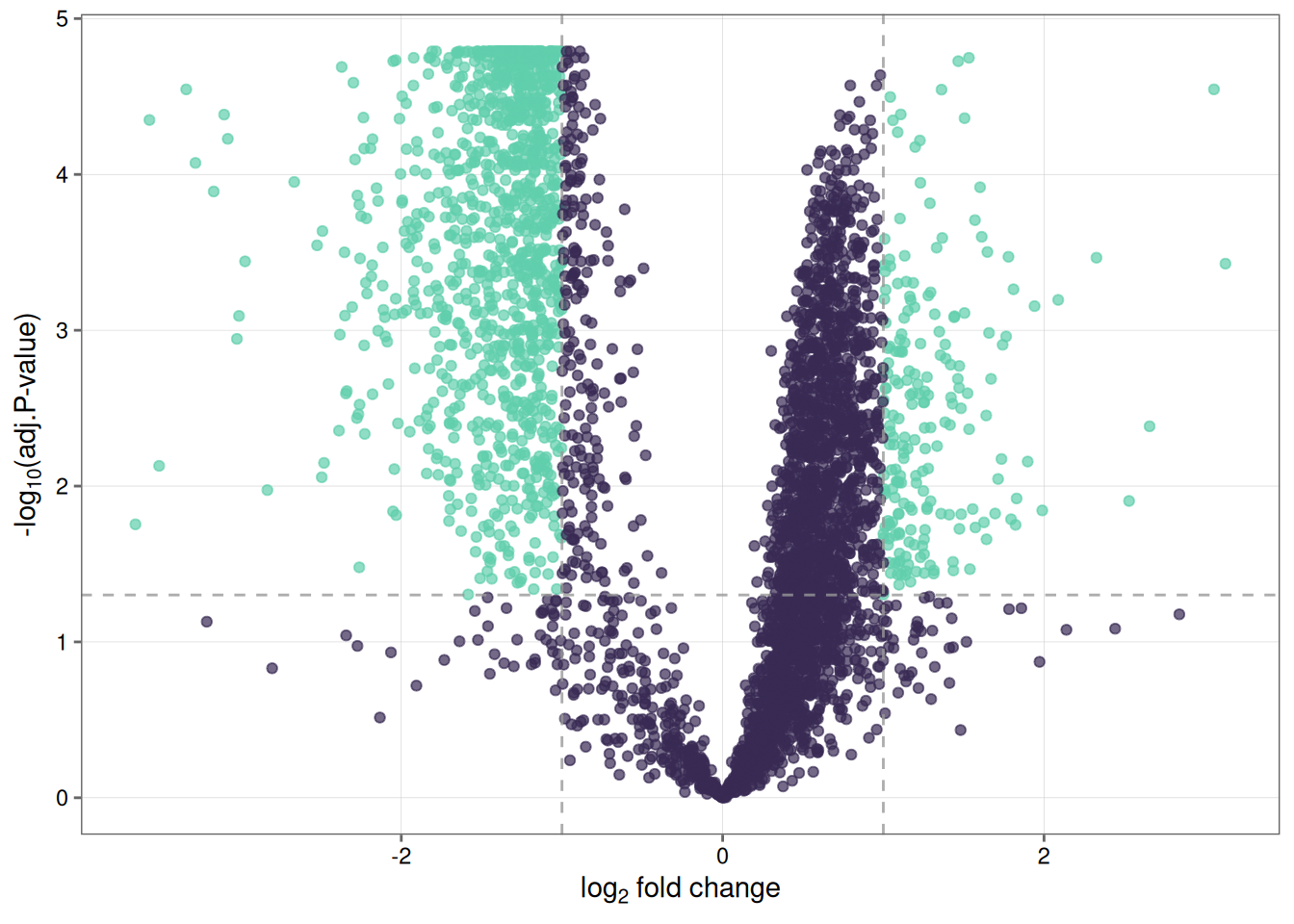### 10. Create a heatmap of differentially expressed proteins

heatmap_de(fit_df, imp_df_mp, palette = "mako")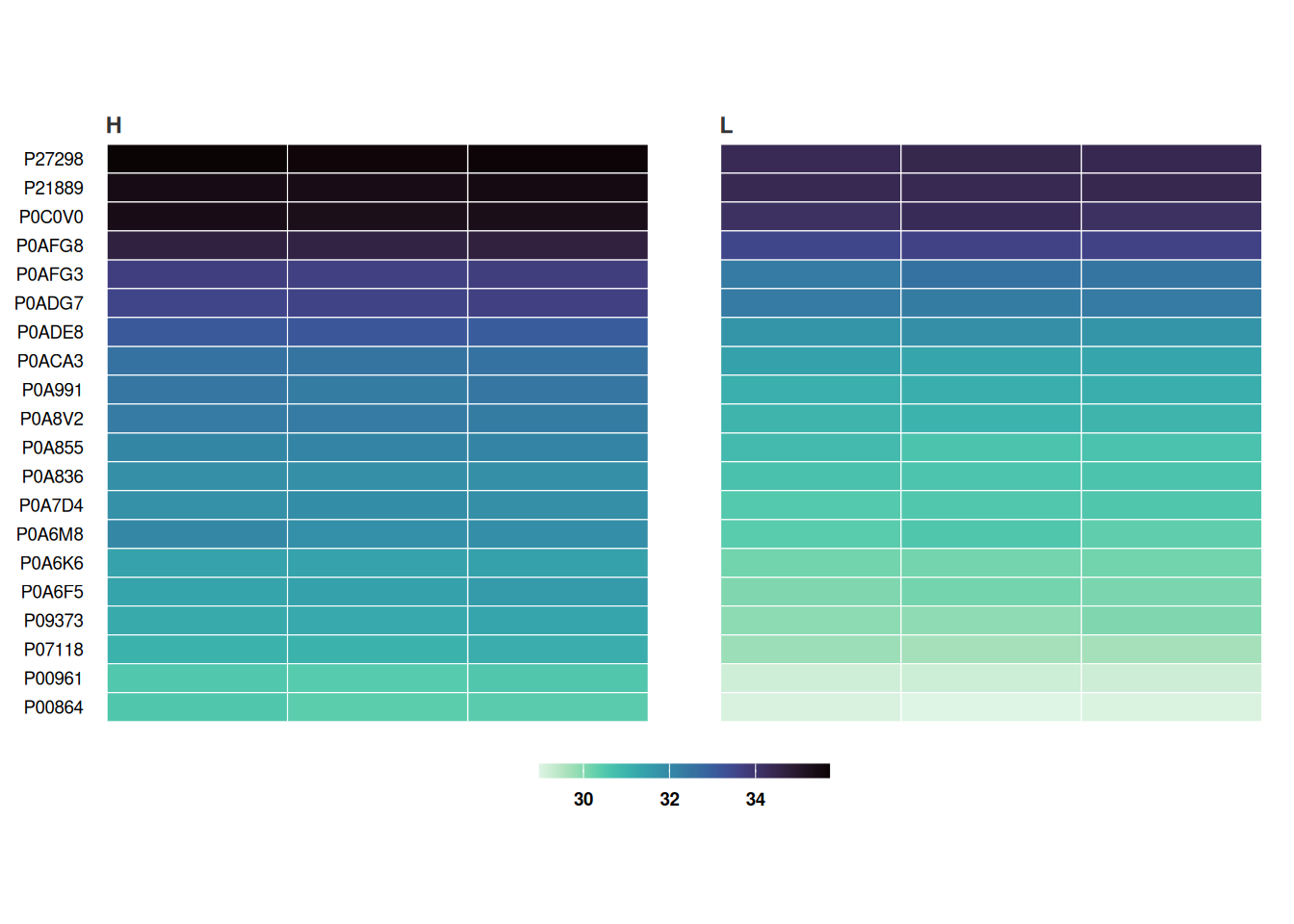Save a copy of the plot in the working directory.

heatmap_de(fit_df, imp_df_mp, palette = "mako", save = TRUE, file_path = ".")